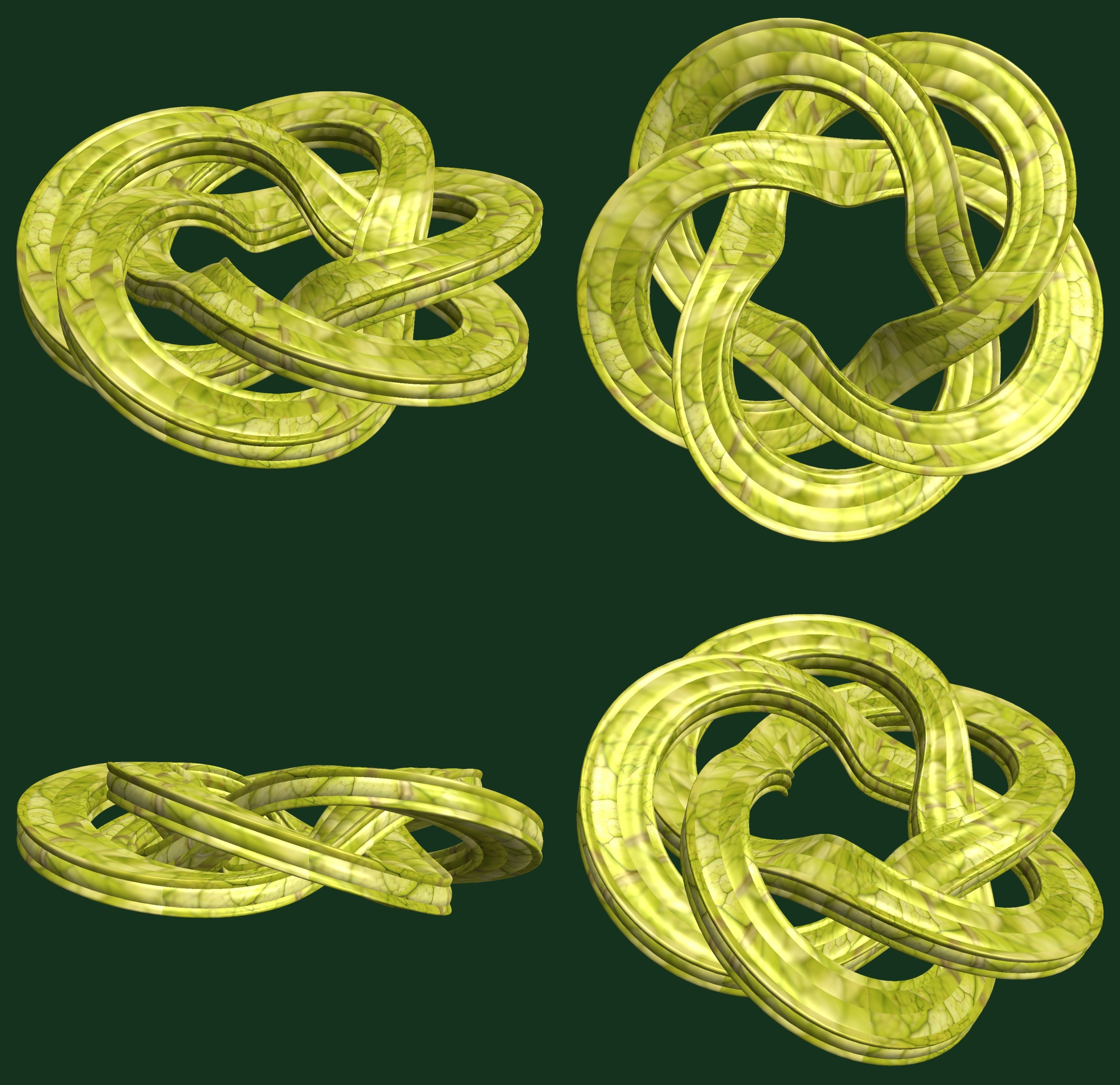# Knot-Tori / 結び目Knot-tori / 結び目 free stock photo

(* === Following code of Mathematica 8 generates this image. === *)

a1 = 10; (* center hole size of a torus *)

a2 = 10; (* center hole size of a torus *)

b1 = 5;(* the number of cross *)

b2 = 3;(* the number of cross *)

b3 = 3;(* the number of waves *)

b4 = 4;(* the number of waves *)

c1 = 0; (* distance from the center of rotation *)

c2 = 0; (* distance from the center of rotation *)

d = 1; (* the number of tori *)

h1 = 2; (* width of a torus *)

h2 = 4; (* width of a torus *)

h3 = 3; (* height of a torus *)

h4 = 1; (* height of a torus *)

SetOptions[

ParametricPlot3D, PlotRange -> Full, Mesh -> None, Boxed -> False, Axes -> False,

PlotPoints -> 400, ImageSize -> 1600, Background -> RGBColor[{20, 50, 30}/255],

PlotStyle -> Directive[Specularity[White, 90], Texture[Import["D:/tmp/71.jpg"]]],

TextureCoordinateFunction -> ({#4, #5 b1 Pi} &), Lighting -> "Neutral"];

x = (a1 - h1 Cos[b3 t] + h2 Sin[b1 s]) Cos[b2 s] + c1;

y = (a2 - h1 Cos[b3 t] + h2 Sin[b1 s]) Sin[b2 s] + c1;

z = h3 Cos[b1 s] + h4 Sin[b4 t] + c2;

rm = Table[{x, y, z}.RotationMatrix[2 Pi (i/d), {0, 1, 1}], {i, d}];

ParametricPlot3D[rm, {t, 0, 2 Pi}, {s, 0, 2 Pi}]

(*---

Another shapes and colors

*)

Free high resolution images knot-tori / 結び目, 3d, トーラス, むすびめ, もよう, りんかん.
Licence: Creative Commons - CC0. Photos transferred to the public domain from Creative Commons.

###### Tags: